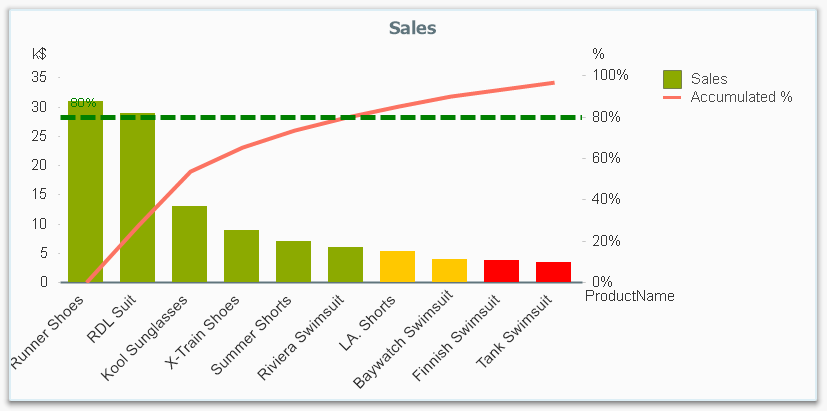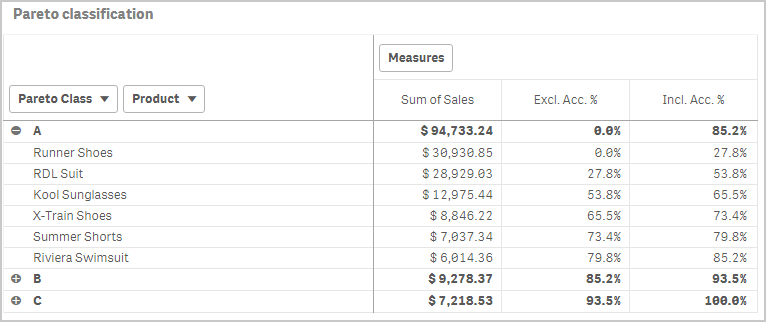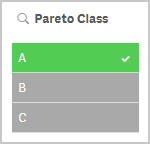# Qlik Design Blog

All about product and Qlik solutions: scripting, data modeling, visual design, extensions, best practices, etc.

Announcements
QlikWorld Online 2021, May 10-12: Our Free, Virtual, Global Event REGISTER TODAYEmployee

## Recipe for a Pareto Analysis – Revisited

“Which products contribute to the first 80% of our turnover?”

This type of question is common in all types of business intelligence. I say “type of question” since it appears in many different forms: Sometimes it concerns products, but it can just as well concern any dimension, e.g. customer, supplier, sales person, etc. Further, here the question was about turnover, but it can just as well be e.g. number of support cases, or number of defect deliveries, etc.It is called Pareto analysis or ABC analysis and I have already written a blog post on this topic. However, in the previous post I only explained how to create a measure which showed the Pareto class. I never showed how to create a dimension based on a Pareto classification – simply because it wasn’t possible.

But now it is.

But first things first. The logic for a Pareto analysis is that you first sort the products according to their sales numbers, then accumulate the numbers, and finally calculate the accumulated measure as a percentage of the total. The products contributing to the first 80% are your best, your “A” products. The next 10% are your “B” products, and the last 10% are your “C” products. In the above graph, these classes are shown as colors on the bars.

The previous post shows how this can be done in a chart measure using the Above() function. However, if you use the same logic, but instead inside a sorted Aggr() function, you can achieve the same thing without relying on the chart sort order. The sorted Aggr() function is a fairly recent innovation, and you can read more about it here.

The sorting is needed to calculate the proper accumulated percentages, which will give you the Pareto classes. So if you want to classify your products, the new expression to use is

=Aggr(
If(Rangesum(Above(Sum({1} Sales)/Sum({1} total Sales),1,RowNo()))<0.8, 'A',
If(Rangesum(Above(Sum({1} Sales)/Sum({1} total Sales),1,RowNo()))<0.9, 'B',
'C')),
(Product,(=Sum({1} Sales),Desc))
)

The first parameter of the Aggr() – the nested If()-functions – is in principle the same as the measure in the previous post. Look there for an explanation.

The second parameter of the Aggr(), the inner dimension, contains the magic of the sorted Aggr():

(Product,(=Sum({1} Sales),Desc))

This structured parameter specifies that the field Product should be used as dimension, and its values should be sorted descending according to Sum({1} Sales). Note the equals sign. This is necessary if you want to sort by expression.

So the Products inside the Aggr() will be sorted descending, and for each Product the accumulated relative sales in percent will be calculated, which in turn is used to determine the Pareto classes.

The set analysis {1} is necessary if you want the classification to be independent of the made selection. Without it, the classification will change every time the selection changes. But perhaps a better alternative is to use {\$<Product=>}. Then a selection in Product (or in the Pareto class itself) will not affect the classification, but all other selections will.

The expression can be used either as dimension in a chart, or in a list box. Below I have used the Pareto class as first dimension in a pivot table.If you use this expression in a list box, you can directly select the Pareto class you want to look at.The other measures in the pivot table are the exclusive and inclusive accumulated relative sales, respectively. I.e. the lower and upper bounds of the product sales share:

Exclusive accumulated relative sales (lower bound):

=Min(Aggr(
Rangesum(Above(Sum({1} Sales)/Sum({1} total Sales),1,RowNo())),
(Product,(=Sum({1} Sales),Desc))
))

Inclusive accumulated relative sales (upper bound):

=Max(Aggr(
Rangesum(Above(Sum({1} Sales)/Sum({1} total Sales),0,RowNo())),
(Product,(=Sum({1} Sales),Desc))
))

Good luck in creating your Pareto dimension!

HIC

Further reading related to this topic:

The sortable Aggr function is finally here!

Recipe for a Pareto Analysis

Recipe for an ABC Analysis

66 CommentsContributor II

Hello Everyone,

As per the discussed logic by hic over the post Recipe for a Pareto Analysis – Revisited‌, want to have a solution over the extended version of the problem which I have mentioned in given below link , So please have a look over it.

Waiting for solution over the given requirement,

Thanks in advance

Aman

https://community.qlik.com/docs/DOC-19616

1,325 ViewsContributor III

Ciao,

i'm encountering an issue with this formula, maybe due to my old 11.2 version?

=Aggr(

If(Rangesum(Above(Sum({1} GIACENZA_ATTUALE*COSTO_MEDIO)/Sum({1} total GIACENZA_ATTUALE*COSTO_MEDIO),1,RowNo()))<0.8, 'A',

If(Rangesum(Above(Sum({1} GIACENZA_ATTUALE*COSTO_MEDIO)/Sum({1} total GIACENZA_ATTUALE*COSTO_MEDIO),1,RowNo()))<0.9, 'B',

'C')),

(COD_ARTICOLO,(=Sum({1} GIACENZA_ATTUALE*COSTO_MEDIO),Desc))

)thank in advance

1,325 ViewsCreator III

Ciao Fabio,

yes, the problem is QV 11.20, you can't use the same parameter for Aggr function.

QV 11.20

Aggr ([ distinct | nodistinct ] [{set_expression}] expression {, dimension})

QV 12.10

Aggr({SetExpression}[DISTINCT] [NODISTINCT ] expr, StructuredParameter{, StructuredParameter})

Regards

Luca Jonathan Panetta

1,325 ViewsContributor III

Ciao,

i have tried this sintax but it wrong.

sorry but i'm not familiar with this type of formulas:

=Aggr(

If(Rangesum(Above(Sum( GIACENZA_ATTUALE*COSTO_MEDIO)/Sum( total

GIACENZA_ATTUALE*COSTO_MEDIO),1,RowNo()))<0.8, 'A',

If(Rangesum(Above(Sum( GIACENZA_ATTUALE*COSTO_MEDIO)/Sum(

total GIACENZA_ATTUALE*COSTO_MEDIO),1,RowNo()))<0.9, 'B',

'C'))

{, COD_ARTICOLO ,(=Sum({1} GIACENZA_ATTUALE*COSTO_MEDIO),Desc)})

Fabio Cuniberto

Da: Luca Jonathan Panetta <qcwebmaster@qlikview.com>

Per: fabio cuniberto <f.cuniberto@arol.it>,

Data: 14/02/2018 09.57

Oggetto: Re: - Recipe for a Pareto Analysis –

Revisited

Recipe for a Pareto Analysis – Revisited

new comment by Luca Jonathan Panetta - View all comments on this blog post

Ciao Fabio,

yes, the problem is QV 11.20, you can't use the same parameter for Aggr

function.

QV 11.20

Aggr ( distinct expression {,

dimension})

QV 12.10

Aggr([DISTINCT] expr, StructuredParameter{,

StructuredParameter})

Regards

Luca Jonathan Panetta

Reply to this email to respond to Luca Jonathan Panetta's comment.

Following Recipe for a Pareto Analysis – Revisited in these streams: Inbox

© 1993-2017 QlikTech International AB | Copyright & Trademarks | Privacy |

Terms of Use | Software EULA

1,325 ViewsCreator III

Hi Fabio,

this is yours expression, in bold I mark the possible errors {, ....  }

=Aggr(

If(Rangesum(Above(Sum( GIACENZA_ATTUALE*COSTO_MEDIO)/Sum( total GIACENZA_ATTUALE*COSTO_MEDIO), 1, RowNo() ))<0.8, 'A',

If(Rangesum(Above(Sum( GIACENZA_ATTUALE*COSTO_MEDIO)/Sum(total GIACENZA_ATTUALE*COSTO_MEDIO),1,RowNo()))<0.9, 'B',

'C'))

{, COD_ARTICOLO ,(=Sum({1} GIACENZA_ATTUALE*COSTO_MEDIO),Desc)})

try this

=Aggr(

If(Rangesum(Above(Sum( GIACENZA_ATTUALE*COSTO_MEDIO)/Sum( total GIACENZA_ATTUALE*COSTO_MEDIO), 1, RowNo() ))<0.8, 'A',

If(Rangesum(Above(Sum( GIACENZA_ATTUALE*COSTO_MEDIO)/Sum(total GIACENZA_ATTUALE*COSTO_MEDIO),1,RowNo()))<0.9, 'B',

'C')),

( COD_ARTICOLO, (=Sum({1} GIACENZA_ATTUALE*COSTO_MEDIO), Desc))

)

Regards

Luca Jonathan Panetta

1,325 ViewsContributor III

this isn't work in Qlik Sense, would anyone can develop a expression for Qlik sense,

also it would be great if we can add time line for calculation. eg, in a period of sales what's the top80 bottom 20

1,325 Views